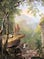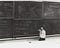Asher Brown Durand’s 𝘒𝘪𝘯𝘥𝘳𝘦𝘥 𝘚𝘱𝘪𝘳𝘪𝘵𝘴 (1849), a classic Transcendentalist painting that puts great emphasis on natural beauty and the meekness of man.

# The Right Philosophy for Our Times

## How Transcendentalism can revitalize our society’s love for the environment

Breathing in the crisp autumn air and staring out over what has been his home, neighborhood, close friend, and therapist, for the past two years, Henry David Thoreau lightly closed the fragile cedar-wooded door of his cabin for the last time.Richard Feynman was a master of learning and the patience that deep understanding requires. His depth of knowledge was exactly what made him such a brilliant teacher. US Department of Energy.

# On Learning Deeply

A few years ago, I remember being faced with an introductory physics problem. It was the first time I had encountered the idea of acceleration, and I was puzzled by the notation for it.

# Linear Algebra 5: Solving Ax = b in non-invertible, non-square matrices

This is a continuation of my Linear Algebra series, which should be viewed as an extra resource while going along with Gilbert Strang’s class 18.06 on OCW. This can be closely matched to Lecture 8 in his series.

Here, we continue our discussion about solving linear systems with elimination. In my Gaussian Elimination series, we explored how square, invertible matrices can be solved by method of elimination and row exchanges — but we never delved into solving rectangular, non-invertible systems.

In the last lesson, we explored how non-square systems can be solved by using Gaussian elimination. …

# Linear Algebra 4: Reduced Row Echelon Form for solving Ax = 0

This is a continuation of my Linear Algebra series, which should be viewed as an extra resource while going along with Gilbert Strang’s class 18.06 on OCW.

Let’s introduce a small additional concept in linear algebra — this is the Reduced Row Echelon Form of a matrix. I’d highly recommend reading the article before this, which details how to solve Ax = 0 in general.

Leaving right where we left off, we eliminated through our A matrix to get something in echelon form, symbolized by the variable U. …

# Linear Algebra 3: Solving Ax = 0, Free Variables, and Pivot Variables, and Echelon Form

## Last time we explored the null space, but how do we find the null space vectors? Additionally, how do we do these on nonsquare matrices?

This article assumes a base knowledge of all basic matrix and vector manipulation concepts, like dot products, linear combinations, as well as ideas involving span, vector spaces and subspaces, and column spaces.

Continuing off the last article, we introduced ideas of the null space.

To review, the null space is the vector space of some group of x that satisfy Ax = 0.

# Linear Algebra 2: Null Space

## Let’s explore the solutions to Ax = 0.

Now that we have explored the column space, we can explore the other vector subspace that matrices can offer, which is the null space. First, we must be familiar with the matrix way of representing a system of equations. I cover this in the first part of my gaussian elimination tutorial.

The entire concept of null space is rather simple. We are trying to find all possible x in a matrix equation Ax = 0. A combination of all these x will form their own subspace, this time in R^n. Let’s walk through all of that .

Let’s start off with an example, in the language of (3, 3) matrices. Let’s also put these in Ax = 0 form, since we’re solving for x. …

# Linear Algebra 1: Vector Spaces and The Column Space

## Delving into real linear algebra with column space and vector spaces

This article assumes knowledge of Gaussian Elimination, matrix multiplication, linear combinations, and a few other concepts. If Gaussian Elimination seems foreign, I have a series of three articles walking through the meanings and mechanics of it.

Now that we have fundamentally covered the elimination way of looking at a system of equations Ax = b, we can delve a little more into the abstract concepts of linear algebra that underlie the system.

We can analyze the rows and columns of our matrix A to understand the concept of vector spaces and subspaces.

A vector space, and later, subspaces, are a group of vectors that are closed under scalar multiplication and addition.

# Matrix Transposes and Symmetric Matrices

## Everything you need to know about transposing matrices and symmetric matrices

Matrix transposes and symmetric matrices are linked — in fact, the definition of a symmetric matrix is that a symmetric matrix A’s transpose gives back the same matrix A.

This is a continuation of my linear algebra series, tied with the 18.06 MIT OCW Gilbert Strang course on introductory linear algebra. Let’s jump right in with the basics on transposes.

We transpose a two-dimensional matrix by using it’s rows as columns, or inversely, as it’s columns as rows. That is all it takes to do the simple operation.

# A Complete Guide to Gaussian Elimination

## Part 3: Factorizations of Gaussian Elimination; A = LU; the way computers execute elimination

In the last article, we discussed how we could express Gaussian Elimination, in terms of matrix multiplications, and how we could multiply those elementary matrices into one single matrix E, which would bring A to the upper triangular U.

Let’s jump right in.

Let’s take a look again at what our example matrix E (our multiple of our three individual elimination matrices) looked like in the last article.

# [2/3] A Complete Guide to Gaussian Elimination

## Part 2 of 3: How we express elimination and row exchanges as matrix multiplications.

We covered all the base concepts for Gaussian Elimination in the first entry in this miniseries on Gaussian Elimination. This article covers how we utilize Gaussian elimination to turn our coefficient matrix A into an upper triangular U so that we can employ back-substitution to solve the system. We also explored the singular cases that can come out of it.

This article series can be thought of as a companion to prof. Gilbert Strang’s course 18.06 on Linear Algebra — more specifically, the first four lectures.

There are two main operations in elimination. These are the elimination operation (the combined multiplication/subtraction step) and row exchanges.